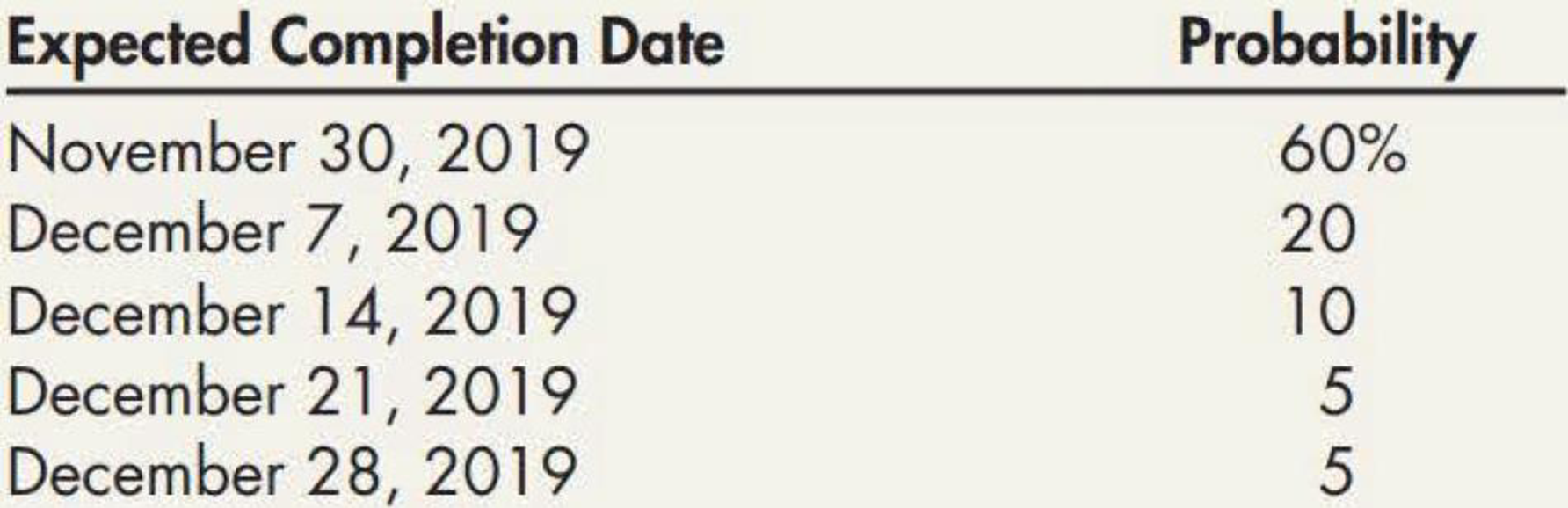Chapter 17, Problem 13E### Intermediate Accounting: Reporting...

3rd Edition
James M. Wahlen + 2 others
ISBN: 9781337788281

#### Solutions

Chapter
Section### Intermediate Accounting: Reporting...

3rd Edition
James M. Wahlen + 2 others
ISBN: 9781337788281
Textbook Problem
1 views

# On March 1, 2019, Elkhart enters into a new contract to build a specialized warehouse for $7 million. The promise to transfer the warehouse is determined to be a performance obligation. The contract states that if the warehouse is usable by November 30, 2019, Elkhart will receive a bonus of$600,000. For every week after November 30 that the warehouse is not usable, the bonus will decrease by \$150,000. Elkhart provides the following completion schedule:Required: 1. Assume that Elkhart uses the expected value approach. What amount should Elkhart use for the transaction price? 2. Assume that Elkhart uses the most likely amount approach. What amount should Elkhart use for the transaction price? 3. Next Level What is the purpose of assessing whether a constraint on the variable consideration exists?

1.

To determine

State the amount used by Company E for the transaction price under expected value approach.

Explanation

Transaction price:

Transaction price is the amount of consideration that is estimated by the company to be authorized in exchange, for delivering the promised goods and services to the customer. Transaction price is examined by the seller by analyzing the terms of the contract and the normally conducts of the business.

Expected value approach: Expected value approach is one of the methods used to evaluate the variable consideration. In expected value approach, a company must recognize the range of probable outcomes and the probabilities connected with each outcome. Then, the expected value is computed as the sum of the “probability-weighted amounts” in this range.

Calculate the expected level of bonus:

 60% × 600,000 = 360,000 20% × 450,000 = 90,000 10% × 300,000 = 30,000 5% × 150,000 = 7,500 5% × 0 = 0 487,500

Table (1)

In this case, the expecte...

2.

To determine

State the amount used by Company E for the transaction price most likely amount approach

3.

To determine

Mention the purpose of assessing whether a constraint on the variable consideration exists.

### Still sussing out bartleby?

Check out a sample textbook solution.

See a sample solution

#### The Solution to Your Study Problems

Bartleby provides explanations to thousands of textbook problems written by our experts, many with advanced degrees!

Get Started